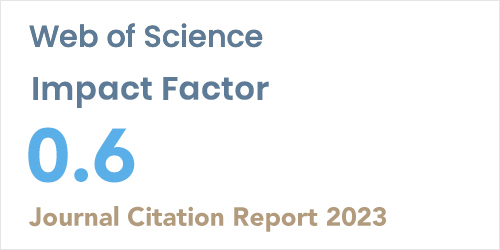ISSN: 1304-7191 | E-ISSN: 1304-7205
Volume: 41 Issue: 4 Year: 2023Modeling and optimization of zinc recovery from enyigba sphalerite in a binary solution of acetic acid and hydrogen peroxide
1Dept. of Chemical Engineering, Madonna University, Akpugo, NIGERIA
2Department of Chemical Engineering, Nnamdi Azikiwe University, Awka, NIGERIA
Sigma J Eng Nat Sci 2020; 38(2): 589-601

#### Abstract

This work focused on the modeling and optimization of zinc recovery from sphalerite in a binary solution of acetic acid and hydrogen peroxide. The sphalerite sample was characterized using X-ray fluorescence (XRF), X-ray diffraction (XRD) and Scanning electron micrograph (SEM). The result revealed that the ore exists as zinc sulphide (ZnS). Levenberg-Marquardt (LM) back-propagation algorithm was employed for artificial neural network (ANN) modeling while central composite rotatable design (CCRD) was deployed for response surface methodology (RSM) modeling. RSM modeling gave optimum conditions of 90oC leaching temperature, 6M acid concentration, 540 rpm stirring rate, 120 minutes leaching time and 6M hydrogen peroxide concentration; at which about 89.91% zinc was recovered. Comparison of the two modeling techniques revealed that ANN (root mean square error, RMSE = 0.530, absolute average deviation, AAD = 0.681, coefficient of determination = 0.996) gave better predictions than RSM (root mean square error, RMSE = 0.755, absolute average deviation, AAD = 0.841, coefficient of determination = 0.991). Hence, ANN demonstrated higher predictive capability than RSM.